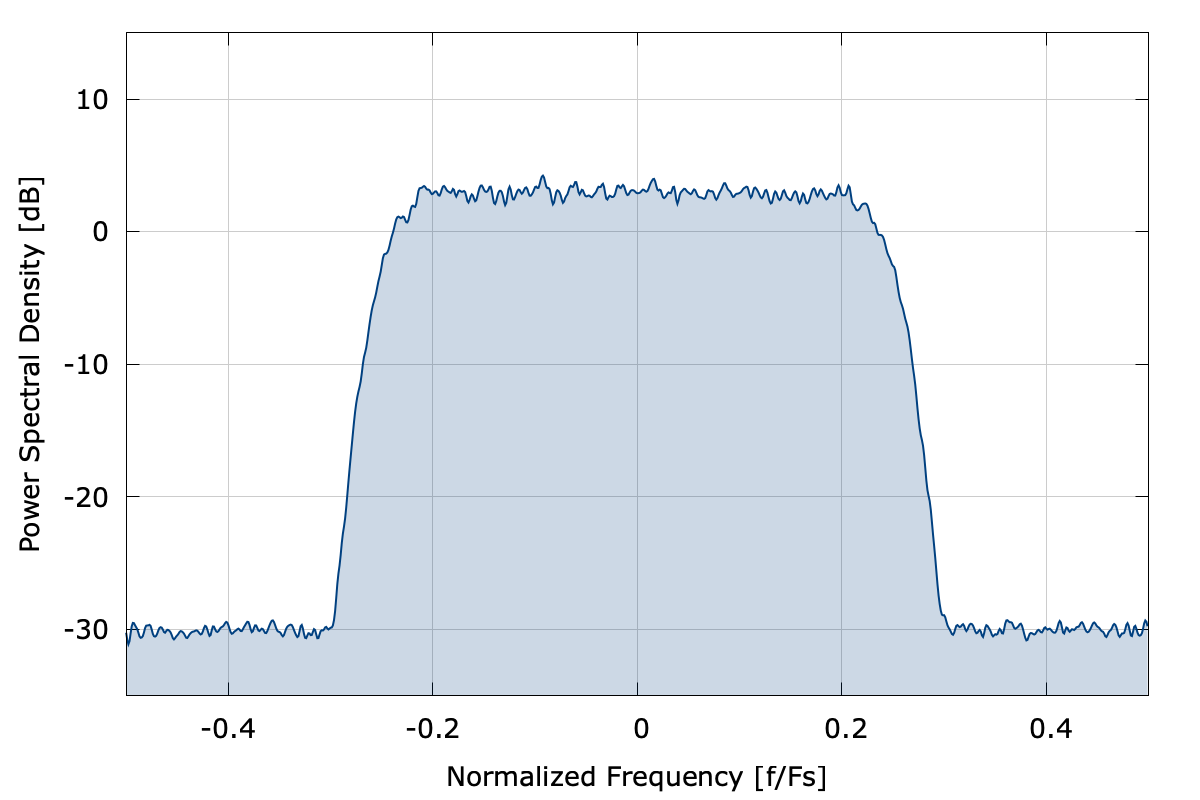# channel

The channel family of objects add signal impairments due to various channel conditions commonly found in wireless communications systems, including

• additive white Gauss noise (AWGN)
• channel gain
• carrier phase and frequency offsets

Shown below is an example of the channel objectFigure [fig-channel-time]. channel output constellationFigure [fig-channel-freq]. Power spectral density

Figure [fig-channel]. channel example of QPSK

Here is a basic example program demonstrating how to use the object:

#include <liquid/liquid.h>

int main() {
// sample buffer
unsigned int  buf_len = 256;    // number of input samples
float complex buf_in [buf_len]; // complex input
float complex buf_out[buf_len]; // output buffer
unsigned int  num_written;      // number of values written to buffer

// create channel object
channel_cccf channel = channel_cccf_create();

// additive white Gauss noise impairment
float noise_floor   = -60.0f;   // noise floor [dB]
float SNRdB         =  30.0f;   // signal-to-noise ratio [dB]

// carrier offset impairments
float dphi          =   0.00f;  // carrier freq offset [radians/sample]
float phi           =   2.1f;   // carrier phase offset [radians]

// multipath channel impairments
float complex* hc   = NULL;     // defaults to random coefficients
unsigned int hc_len = 4;        // number of channel coefficients

float sigma         = 1.0f;     // standard deviation for log-normal shadowing
float fd            = 0.1f;     // relative Doppler frequency

// print channel internals
channel_cccf_print(channel);

// fill buffer and repeat as necessary
{
// apply channel to input signal
channel_cccf_execute_block(channel, buf_in, buf_len, buf_out);
}

// destroy channel
channel_cccf_destroy(channel);
}


For a more detailed example, refer to examples/channel_cccf_example.c located under the main liquid project source directory.